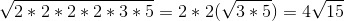# PSAT Math : How to multiply square roots

## Example Questions

### Example Question #181 : Arithmetic

Multiply and simplify. Assuming all integers are positive real numbers.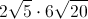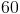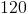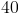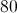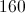Explanation:Multiply the coefficents outside of the radicals.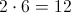Then multiply the radicans. Simplify by checking for a perfect square.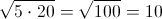Final answer is your leading coefficent,, multiplied by the answer acquired by multiplying the terms under the radican,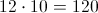The final answer is.

### Example Question #11 : Basic Squaring / Square Roots

Mulitply and simplify. Assume all integers are positive real numbers.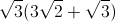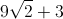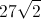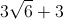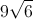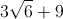Explanation:Order of operations, first distributing the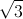to all terms inside the parentheses.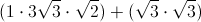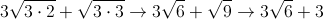The final answer is.

### Example Question #12 : Basic Squaring / Square Roots

The square root(s) of 36 is/are ________.

None of these answers are correct.

-6

6, -6, and 0

6 and -6

6

6 and -6

Explanation:

To square a number is to multiply that number by itself. Because 6 x 6 = 36 AND -6 x -6 = 36, both 6 and -6 are square roots of 36.

### Example Question #13 : Basic Squaring / Square Roots

Simplify: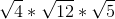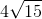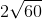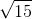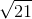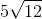Explanation:

Multiplication of square roots is easy! You just have to multiply their contents by each other. Just don't forget to put the result "under" a square root! Therefore:becomes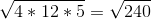Now, you need to simplify this: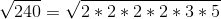You can "pull out" twos.  (Note, that it would be even easier to do this problem if you factor immediately instead of finding out that.)

After pulling out thes, you get: